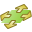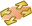# BO Card

This card defines a ground plane with the reflection coefficient approximation (at z = 0). All computations that follow this card will include the ground plane.

On the Home tab, in the Planes / arrays group, click thePlane / ground icon. From the drop-down list, click theReflective ground (BO) icon.

## Parameters:

No reflection coefficient ground
No ground plane. This option is used to switch off the reflection ground if the effect of different grounds are considered in a single input file, for example to consider a GF card.
Reflection coefficient ground plane
Use the reflection coefficient ground plane approximation with the material parameters specified in the remaining input fields.
Perfectly electric conducting ground
Use an ideal electric ground in the plane z = 0. In this case the remaining parameters are ignored.
Perfectly magnetic conducting ground
Use an ideal magnetic ground in the plane z = 0. In this case the remaining parameters are ignored.
Material label
The label of the medium to be used, as defined in the DI card.

It should be noted that it is not possible to calculate the fields below the ground plane (in the z < 0 half space). In addition all structures must be in the region z > 0. If calculations inside the ground are required, for example when there are structures below ground, the exact Sommerfeld integrals (GF card) must be used.

When using a perfect electric or magnetic reflection coefficient ground plane, structures can be arbitrarily close to the ground (while remaining above it). Segment end points and triangle edges lying in the plane of the ground plane will make electrical contact with a perfect electric ground plane. For a perfect magnetic ground plane the boundary condition forces the current to zero at this point.

If real ground parameters are used, the reflection coefficient approximation is more accurate for structures further from the ground plane. Typically structures should not be closer than about $\frac{\lambda }{10}$ will give a warning if this is the case).

A dielectric ground (real earth) can only be used with bodies treated with MoM, MLFMM, PO, FEM, or the hybrid MoM/PO.
Note: The hybrid MoM/UTD method cannot be used in the presence of a real ground.